# Pre Treatment & Physical Treatment: Aeration (Part - 1) Computer Science Engineering (CSE) Notes | EduRev

## Computer Science Engineering (CSE) : Pre Treatment & Physical Treatment: Aeration (Part - 1) Computer Science Engineering (CSE) Notes | EduRev

The document Pre Treatment & Physical Treatment: Aeration (Part - 1) Computer Science Engineering (CSE) Notes | EduRev is a part of the Computer Science Engineering (CSE) Course Environmental Engineering - Notes, Videos, MCQs & PPTs.
All you need of Computer Science Engineering (CSE) at this link: Computer Science Engineering (CSE)

AERATION BASICS

Factors affecting removal of compounds by aeration

• Physico- chemical properties of compound to be removed like hydrophobicity, surface area, etc.
• Temperature of water & air.
• Process parameters for aeration like air to water ratio, available area of mass transfer, contact time, etc.

Calculation of solubility of gases

Henrys’ Law is defined as: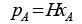(3.3.1)

Where pA is the partial pressure of any compound A in air (atm), H is the Henrys' constant which depends upon temperature and xis the mol fraction of compound A in water. By definition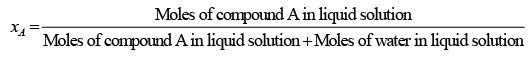Since moles of oxygen in liquid solution are usually very less as compared to moles of water in liquid solution, therefore,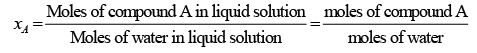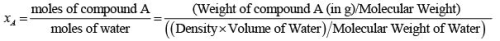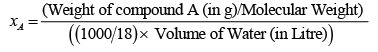(3.3.2)

xA = ( ( C oncentration of compound A (in g/L)x 18) / ( M olecular W eight of compound A (in g/mol) x 10 3 ) )

x= ( ( CA (in g/L) 18) ( MW (in g/mol) 103))

Putting in earlier equation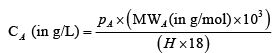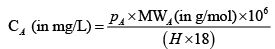(3.3.3)
Where, CA  is the solubility of compound A in water.

Variation of solubility of gases with temperature

Solubility of gases decreases with an increase in temperature. The change in Henrys’ constant with temperature can be computed using van’t Hoff type of equation: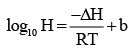(3.3.4)
Where, -ΔH is the Heat of absorption in kcal/kmol, R is the gas constant (=1.987 kcal/K-kmol), T is temperature in K and b is a dimensionless empirical constant.

Problem 3.3.1: The particle pressure of O2 in atmosphere is 0.21 atm. Find the concentration of O2 in water (in mg O2/ litre of water) at 20 oC & 5 oC. Given that for oxygen, Henry’s constant (H) is equal to 4.3×104 atm at 20 oC, ΔH=1.45 103 kcal/kmol, and b=7.11.

Solution: Given that: pA= 0.21 atm, H = 4.3×104 atm at 20 oC.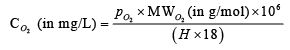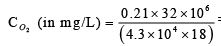= 8.682 mg O2/ litre of water at 20 oC.

Now calculating Henrys’ constant at 5 o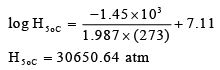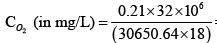= 12.17 mg O2/ litre of water at 5 oC.

AERATION TYPES

• Diffused or Submerged Aeration: Submerged a eration s ystems are used in lakes, reservoirs, and wastewater treatment facilities to increase dissolved oxygen (DO) levels and promote water circulation. Submerged diffusers release air or pure oxygen bubbles at depth, producing a free, turbulent bubble-plume that rises to the water surface through buoyant forces. The ascending bubble plume entrains water, causing vertical circulation and lateral surface spreading. Oxygen transfers to the water across the bubble interfaces as the bubbles rise from the diffuser to the water surface .
• Spray aeration: Spray aeration removes low levels of volatile contaminants. In a spray aeration system, water enters through the top of the unit and emerges through spray heads in a fine mist. Treated water collects in a vented tank below the spray heads. Volatile contaminants are released and vented to the outside .
• Water fall type of aeration: It involves flow of water over media forming droplets or thin film of water so as to contact with air.
Offer running on EduRev: Apply code STAYHOME200 to get INR 200 off on our premium plan EduRev Infinity!

56 docs|57 tests

,

,

,

,

,

,

,

,

,

,

,

,

,

,

,

,

,

,

,

,

,

;